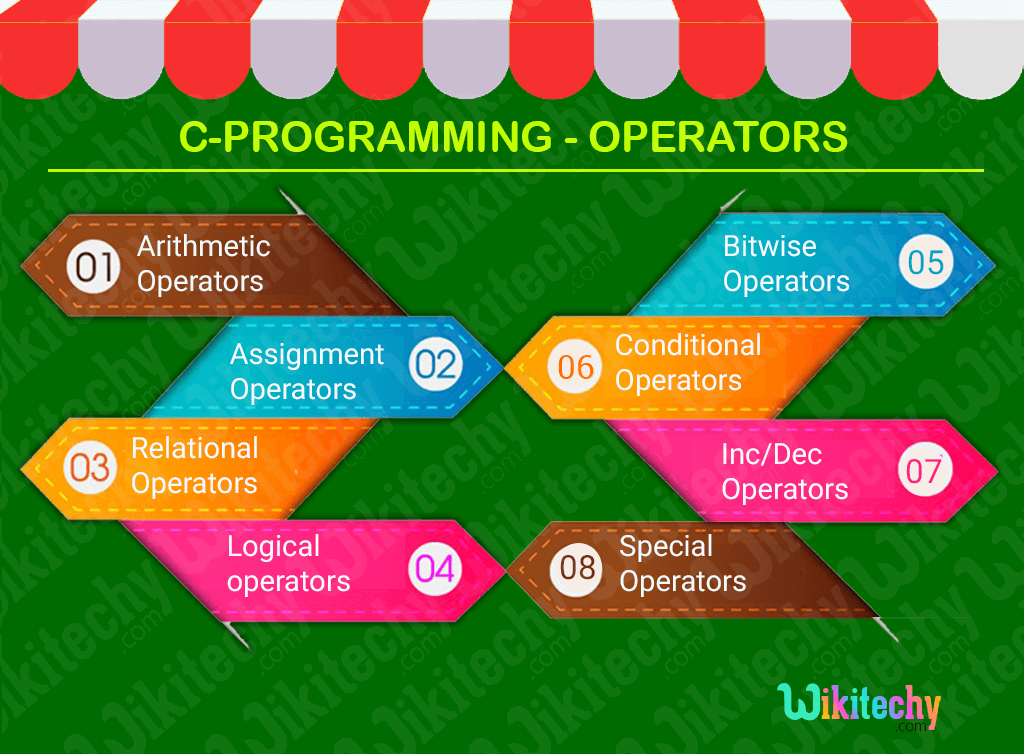# C - Operator

## C - Operator - Definition and Usage

• In C-Programming the symbols are used for logical and mathematical operations called as operators in C.
• Types of operators in C-programming :## Arithmetic operators :

• In C-Programming the Arithmetic operators are used to perform mathematical calculations such as
• Subtraction(-),
• Multiplication(*),
• Division(/) and
• Modulus(%).

## Assignment operators :

• In C programs, values for the variables are assigned using assignment operators (=).

## Relational operators :

• Relational operators are used to find the relation between two variables, i.e. to compare the values of two variables in a C programming.

## Logical operators :

• These operators are used to perform logical operations on the given expressions.
• There are three logical operators in C language. They are,
• logical AND (&&),
• logical OR (||) and
• logical NOT (!)

## Bitwise operators :

• These operators are used to perform bit operations.
• Decimal values are converted into binary values which are the sequence of bits and bit wise operators work on these bits.
• Bit wise operators in C programming are as follows:
• & (bitwise AND),
• | (bitwise OR),
• ~ (bitwise NOT),
• ^ (XOR),
• << (left shift) and
• >> (right shift).

## Conditional operator :

• Conditional operators return one value if condition is true and returns another value is condition is false.
• Conditional operators is also known as ternary operator represented by the symbol “ ? :” (question mark).

## Increment/decrement Operator :

• In C- Programming Increment operators (++) is used to increase the value of the variable by one.
• In C- Programming decrement operators (--) is used to decrease the value of the variable by one.

## Special Operator :

• In c-program some of the operators are used as Special operators they are
• & - operator is used to get the address of the variable;
• * - operator is used as pointer of the variable.
• sizeof() - operator is used to define the size of the variable.